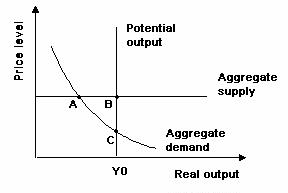### CFA Practice Question

There are 539 practice questions for this study session.

### CFA Practice Question

Refer to the graph below. The economy is in short-run equilibrium at ______.A. point A
B. point B
C. point C

Short-run equilibrium exists at the point at which the aggregate demand curve intersects the aggregate supply curve.